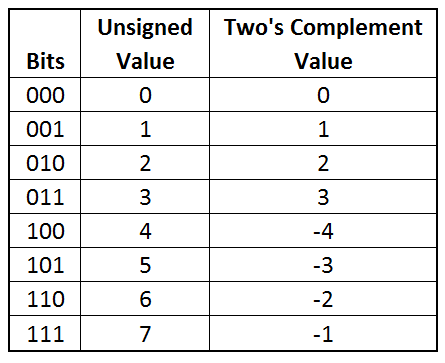# Signed Integer Ranges: Why and How

Whether you are working in T-SQL, Oracle, MySQL, C#, or Java, the range of possible values for a signed (positive or negative) 32-bit integer is from$-2^{31}$ or (-2,147,483,648) to$(2^{31})-1$ or (2,147,483,647). The fact that it's so consistent across so many different platforms (and also against plenty of others I didn't list) means there has to be more to it than just the preference of some developers somewhere, right? Exactly right.

## Down to the bits

Any data your computer deals with, be it numbers, text, music or videos, all end up in binary at one point or another. Binary means values are in "base 2", where each digit represents a power of 2, and the possible values for that digit are 0 or 1. A digit capable of storing only the values 0 and 1 is typically referred to as a bit, which is short for "binary digit". This is in stark contrast to the decimal or "base 10" number system commonly used, where each digit represents a power of 10 and the possible values for that digit are 0 to 9. To show how values are calculated in different number systems, here is the value 37 in both binary and decimal:

I realize that's an extremely brief example, but if you want to learn more about binary numbers, there are tons of great resources online.

But what about negative values? Negative numbers can be represented a bunch of different ways. Sometimes it's done with the minus sign$-150$

or with parentheses$(150)$

but those are all formats, or different ways of expressing a value. A format can be changed without altering the value itself.

How do you really represent that a value is negative? Since binary consists only of bits storing the values 0 and 1, what if the first bit of a binary number served not as a value, but to show whether it was positive or negative? A "1" in the left-most bit would effectively mean the value is negative, and a "0" would mean it is positive. Losing a bit to storing positive or negative would effectively cut the number of values you could store in half (since you are losing a power of two), but it would be awfully handy. There's a little bit more to this method than that, but it's known as…

## Two's Complement

Two's Complement is a great way to express signed numbers in binary, for reasons we will see shortly.

Expressing a negative value via two's complement is a rather simple process. Start with the binary expression of the positive value you want to negate. Next, flip all the bits so all the 0's become 1's, and all the 1's become 0's. If we were to stop here, this would be the one's complement of the value. To get the two's complement, we add 1 to the one's complement. Here's an example for the number 19:

``````1010011  (19, unsigned, with leading 0 added)
2101100  (bits flipped)
3101101  (add 1. This is -19 in two's complement)
``````

We now have the two's complement of 19, which has the value -19.

Why did we have to add one? Let's say we're in a five-bit environment and want to find the two's complement of zero (which would be negative zero). The number zero is represented as 00000 in this case. If we flip each bit, we now have 11111, and by adding one to that we now need a sixth bit so we can arrive at 100000. Since we're still working in a five-bit environment, we ignore the leftmost bit, bringing us back to 00000, which represents both zero and negative zero. Having a single value for positive and negative zero is a key advantage that two's complement has over ones' complement.

An even larger advantage of two's complement is that addition, subtraction, and multiplication work exactly the same as if all values were positive. You can even use the carry method to do this (like you were probably taught in school, but your kids most likely won't learn thanks to common core in the US.) As a demonstration, let's add 24 to the -19 value we just computed. The result should be 5.

And indeed it is! If you're paying close attention you will notice that I truncated the result to 6 bits and ignored the left-most carry. Restricting the result to the same number of significant bits you are adding is a requirement to arrive at a correct answer.

This is really neat, but it still doesn't answer our original question about the possible range of values. Let's look at the values you can create with 3 bits.Three bits allow 8 or$2^3$ values to be stored, and in the case of unsigned numbers, those values are from 0 to 7.  The two's complement values of those same bit arrangements gives a range of -4 to 3.

Thinking of it in powers of 2, three bits in two's complement allows us to store values from$-(2^2)$ to$(2^2)-1$ . Both the exponents are 2 instead of 3 because a bit is being used to determine whether the value is positive or negative. If we showed 2 as one less than three, the value range would look like:$-(2^{(3-1)})$ to$2^{(3-1)}-1$

So for$n$ bits, two's complement lets you express values ranging from$-(2^{(n-1)})$ to$2^{(n-1)}-1$ . This is exactly why for a 32-bit integer, the range is$-(2^{31})$ to$2^{31}-1$ .

And there you have it. Two's complement is the reason why basically any signed data types have the range that they do.